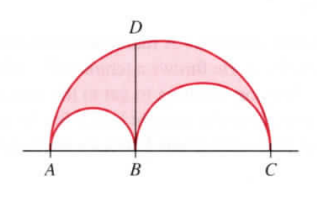×
Get Full Access to Calculus: Early Transcendentals - 1 Edition - Chapter 4.4 - Problem 54e
Get Full Access to Calculus: Early Transcendentals - 1 Edition - Chapter 4.4 - Problem 54e

×

# The arbelos An arbelos is the region enclosed by threeISBN: 9780321570567 2

## Solution for problem 54E Chapter 4.4

Calculus: Early Transcendentals | 1st Edition

• Textbook Solutions
• 2901 Step-by-step solutions solved by professors and subject experts
• Get 24/7 help from StudySoup virtual teaching assistantsCalculus: Early Transcendentals | 1st Edition

4 5 1 271 Reviews
24
2
Problem 54E

An arbelos is the region enclosed by three mutually tangent semicircles; it is the region inside the larger semicircle and outside the two smaller semicircles (see figure).a. Given an arbelos in which the diameter of the largest circle is 1, what positions of point B maximize the area of the arbelos?

b. Show that the area of the arbelos is the area of a circle whose diameter is the distance BD in the figure.

Step-by-Step Solution:

Solution Step 1 : (a)The given diagram is

Step 2 of 4

Step 3 of 4

## Discover and learn what students are asking

Calculus: Early Transcendental Functions : Multiple Integration
?In Exercises 1 and 2, evaluate the integral. $$\int_{y}^{2 y}\left(x^{2}+y^{2}\right) d x$$

Statistics: Informed Decisions Using Data : Inference about the Difference between Two Medians: Dependent Samples
?In Problems 3–10, use the Wilcoxon matched-pairs signedranks test to test the given hypotheses at the a = 0.05 level of significance. The dependent sa

Unlock Textbook Solution